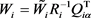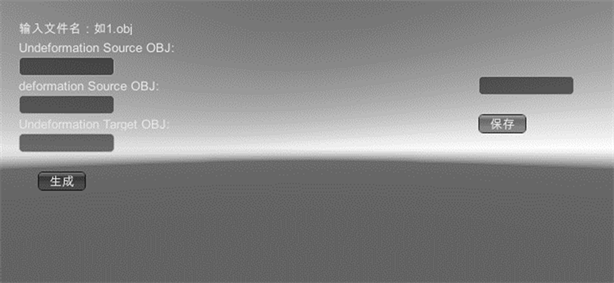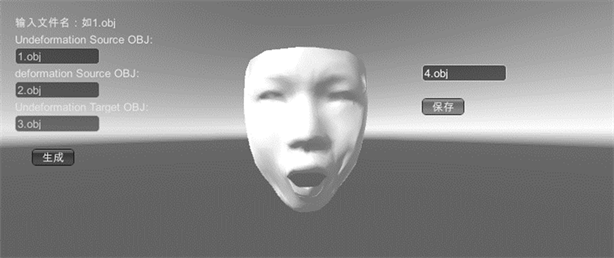基于样例生成面部表情基系统的设计与实现Design and Implementation of Expression Radical Generation System Based on Examples

• 全文下载: PDF(494KB)    PP.682-688   DOI: 10.12677/CSA.2019.94077
• 下载量: 151  浏览量: 205

There are quite a few factors that determine the authenticity of the characters together, and facial expressions undoubtedly occupy a large proportion of them. And, there have been many studies on the generation of facial expressions. One of them is to obtain a target expression by linearly combining multiple facial expressions. That requires a system that can automatically generate facial expression to provide desired expressions. This paper focuses on a sample-based facial expression generation system. It mainly talks about using the affine transformation to represent the deformation of the source triangle mesh, and constrain the deformation mesh through the consistency of affine transformation. The final simulation result shows that the sample-based facial expression generation system can effectively imitate person’s facial expression.

1. 引言

1、量化模板表情的形变

2、使用仿射变换的一致性约束网格形变

2. 生成算法设计

2.1. 计算样例面部表情模型的形变梯度

${V}_{i4}={V}_{i1}+\frac{\left({V}_{i2}-{V}_{i1}\right)×\left({V}_{i3}-{V}_{i1}\right)}{\sqrt{‖\left({V}_{i2}-{V}_{i1}\right)×\left({V}_{i3}-{V}_{i1}\right)‖}}$ (1)

2.2. 计算目标面部表情模型的形变梯度

${F}_{i}{W}_{i}={\stackrel{˜}{W}}_{i}$ (2)

$\begin{array}{l}{W}_{i}=\left[\begin{array}{cc}{v}_{i2}-{v}_{i1}& {v}_{i3}-{v}_{i1}\end{array}\right]\\ {\stackrel{˜}{W}}_{i}=\left[\begin{array}{cc}{\stackrel{˜}{v}}_{i2}-{\stackrel{˜}{v}}_{i1}& {\stackrel{˜}{v}}_{i3}-{\stackrel{˜}{v}}_{i1}\end{array}\right]\end{array}$ (3)

${W}_{i}={Q}_{i}\left[\begin{array}{c}{R}_{i}\\ 0\end{array}\right]=\left[\begin{array}{cc}{Q}_{i\alpha }& {Q}_{i\beta }\end{array}\right]\left[\begin{array}{c}{R}_{i}\\ 0\end{array}\right]={Q}_{i\alpha }{R}_{i}$ (4)(5)

2.3. 三角网格间的映射

$M=\left\{\left({S}_{1},{T}_{1}\right),\left({S}_{2},{T}_{2}\right),\cdots ,\left({S}_{|M|},{T}_{|M|}\right)\right\}$ (6)

2.4. 仿射变换的一致性

${T}_{j}{v}_{i}+{d}_{j}={T}_{k}{v}_{i}+{d}_{k}\text{,}\text{\hspace{0.17em}}\text{\hspace{0.17em}}\text{\hspace{0.17em}}\forall i,\forall j,k\in p\left({v}_{i}\right)$ (7)

$\underset{{T}_{1}+{d}_{1}\cdots {T}_{m}+{d}_{m}}{\mathrm{min}}\underset{i=1}{\overset{m}{\sum }}{‖{S}_{i}-{T}_{i}‖}_{F}^{2}$ (8)

2.5. 确定并优化顶点集合

$\underset{{\stackrel{˜}{V}}_{1}\cdots {\stackrel{˜}{V}}_{n}}{\mathrm{min}}\underset{i=1}{\overset{m}{\sum }}{‖{S}_{i}-{T}_{i}‖}_{F}^{2}$ (9)

Subject to ${\stackrel{˜}{V}}_{j}=c$

$\underset{{\stackrel{˜}{V}}_{1}\cdots {\stackrel{˜}{V}}_{n}}{\mathrm{min}}\underset{i=1}{\overset{|M|}{\sum }}{‖{S}_{{S}_{i}}-{T}_{{T}_{i}}‖}_{F}^{2}$ (10)

Subject to ${\stackrel{˜}{V}}_{j}=c$

3. 系统实现

3.1. 输入模块的实现

3.2. 输出模块的实现

3.3. 图形界面Figure 1. User interface of systemFigure 2. System result of 3D model

4. 结论

  Parke, F.I. (1972) Computer Generated Animation of Faces. Proceedings of the ACM Annual Conference, 1, 451-457. https://doi.org/10.1145/800193.569955  Pyun, H., Kim, Y., Chae, W., et al. (2003) An Exampled-Based Approach for Facial Expression Cloning. Proceedings of the ACM SIGGRAPH/Eurographics Symposium on Computer Animation, Eurographics Associa-tion Press, Aire-la-Ville, 167-176.  Noh, J.Y. and Neumann, U. (2001) Expression Cloning. Proceedings of the 28th Annual Conference on Computer Graphics and Interactive Techniques, ACM Press, New York, 277-288. https://doi.org/10.1145/383259.383290  Escher, M., Pandzic, I. and Magnenat-Thalmann, N. (1998) Facial Deformations for MPEG-4. Proceedings Computer Animation’98 (Cat. No.98EX169), Philadelphia, 8-10 June 1998, 56. https://doi.org/10.1109/CA.1998.681908  Kshirsagar, S., Garchery, S. and Magnenat-Thalmann, N. (2000) Feature Point Based Mesh Deformation Applied to MPEG-4 Facial Animation. In: Magnenat-Thalmann, N. and Thalmann, D., Eds., Deformable Avatars. IFIP—The International Federation for Information Processing, Vol. 68. Springer, Boston, MA24-34.  尹宝才, 王恺, 王立春. 基于MPEG-4的融合多元素的三维人脸动画合成方法[J]. 北京工业大学学报, 2011, 37(2): 266-271.  黄晓钦, 林裕旭, 宋黎明, 等. 非线性联合学习的三维人脸表情合成方法[J]. 计算机辅助设计与图形学学报. 2011, 23(2): 364-370.